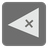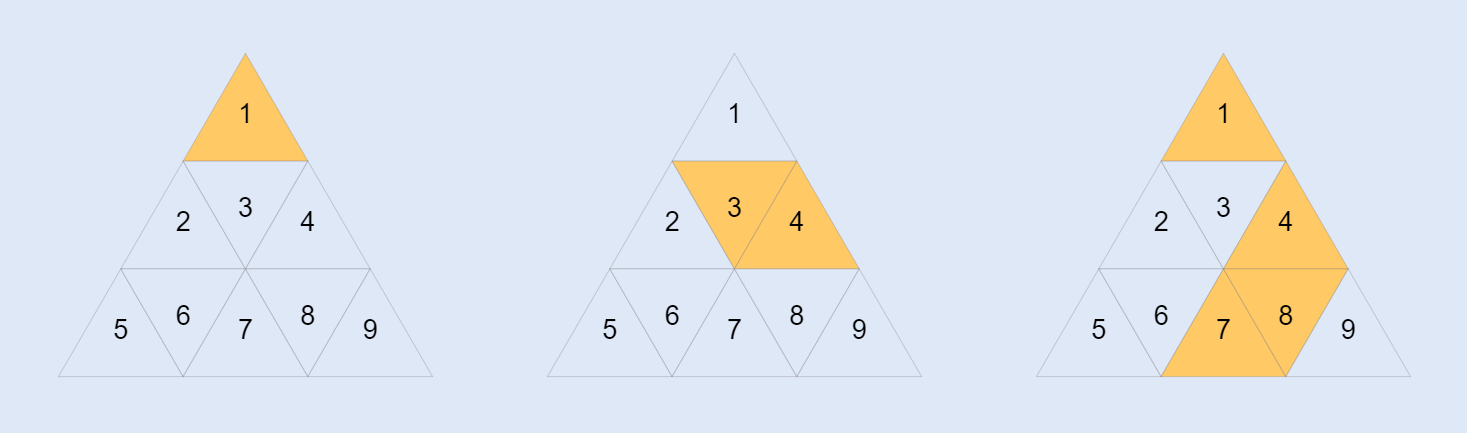Triangular Islands
Undefined
English

The robots have discovered the strange islands in the Bermuda Triangle.
You need to help robots calculate them.

The triangular sea area is divided into several triangular areas, numbered from top to bottom and from left to right in row-major order.

You are given a set of integers with land numbers as input values.

• Triangles with adjacent sides are considered to be a group of the same island.
• The number of those triangles is the island area.

You have to return a list (or an iterable) of the island areas you’ve found.

Example:

```sorted(triangular_islands({1})) == 
sorted(triangular_islands({4, 3})) == 
sorted(triangular_islands({7, 1, 8, 4})) == [1, 3]```Input: A set of integers with land numbers.

Output: A list (or an iterable) of integers with the island areas.

Precondition:

• 1 ≤ land_number ≤ 100